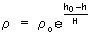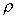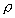# Long-term Orbit Predictor

The Long-term orbit predictor (LOP) allows accurate prediction of the motion of a satellite's orbit over many months or years. The LOP propagator uses the same orbital elements as the Two-Body, J2 and J4 propagators.

The LOP propagator does not propagate its position; therefore, Access, Graphics, some reports, and some Vector Geometry, Time, and Calculation components may not be defined for a Satellite using the LOP propagator.

After choosing the LOP propagator, define its parameters:

1. Get a new initial vector with the Initial State Tool (optional).
2. Set the satellite's Start Time, Stop Time, and Step Size.
3. Choose a Coordinate System.
4. Choose a Coordinate Type and enter values for the orbital parameters.
5. Define the Force Model.

The LOP propagator works in the Mean of Epoch reference frame where the epoch of the reference frame is the epoch of the initial conditions. For the purposes of central body gravity effects, the movement of the Earth's equator due to precession and nutation are ignored (the Earth Fixed equator is assumed to be in alignment with the Mean of Epoch equator). It is therefore often desirable to view the evolution of the orbit plane (inclination and right ascension of the ascending node) in Mean of Epoch coordinates.

While LOP accepts inputs in osculating elements, STK converts those inputs to mean elements before propagating an orbit. This is an iterative process that ends based on internal convergence criteria. After the mean elements are integrated, they are transformed back to osculating elements for output. Since the conversion to mean elements is not exact, it is possible for the initial state output by LOP to be slightly different from the original initial state.

##### Defining a LOP Force Model

The Force Models... button on the Orbit page allows you to set advanced options for the LOP propagator.

Earth Gravity

OptionDescription
Maximum DegreeThe maximum degree of geopotential coefficients to be included for Earth gravity computations. Valid range is from 2 to 70.
Maximum OrderThe maximum order of Geopotential coefficients to be included for Earth gravity computations. Valid range is from 0 to the Maximum Degree.

Third Body Gravity

OptionDescription
Use Solar GravityIf ON, the effects of solar gravity on the satellite are modeled.
Use Lunar GravityIf ON, the effects of lunar gravity on the satellite are modeled.

Drag

OptionDescription
UseIf ON, the drag parameters set here are modeled.
CdThe atmospheric drag coefficient.
Advanced Settings...Click Advanced Settings... to define further the drag model to be used during LOP calculations.

OptionDescription
UseIf ON, solar radiation pressure values entered here are modeled.
Atmosphere HeightHeight of atmosphere blockage used when calculating shadow entry and exit in solar radiation computations.

Physical Data

OptionDescription
Drag Cross-Sectional AreaThe satellite's cross-sectional area to be used in atmospheric drag calculations.
SRP Cross-Sectional AreaThe satellite's cross-sectional area to be used in solar radiation pressure calculations.
Satellite MassThe mass of the satellite to be used in atmospheric drag and solar radiation pressure calculations.

Use the Advanced Settings... button in the LOP Force Model window to define further the drag model to be used for calculations.

Atmospheric Density Models

ModelDescription
1976 Standard Atmosphere ModelUses a table of densities as a function of altitudes to calculate atmospheric density.
Exponential Density ModelUses the following equation to calculate the atmospheric density at any given altitude:where:
•equals the density at the user-specified altitude
• h equals the user-specified altitude
•O equals the reference density
• hO equals the reference height
• H equals the scale height

Atmospheric density model options

OptionDescription
Use Osculating AltitudeIf ON, a short period variation due to J2 perturbations is used when calculating altitude.
Maximum Drag AltitudeThe altitude above which drag calculations are not considered.
Density Weighting FactorThe scale factor to be used during density calculations.

If the Atmospheric Density Model is Exponential Density, define the following parameters:

OptionDescription
Reference DensityO in the above equation.
Reference HeighthO in the above equation.
Scale HeightH in the above equation.